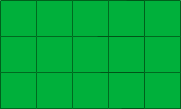Name: Craig Who is asking: Parent Level: Middle Question: my parents want to lay sod in our back yard. the sod cost \$2.00 per square foot. The size of our yard is 20x20 and 26x41 how do i figure out what the square foot is and how much will this cost? Hi Craig, Imagine that you buy the sod in squares that are one foot by one foot, each costing \$2.00, and you want to cover the 20 foot by 20 foot piece of the yard. You start in a corner, as in the diagram below.The diagram has 3 rows of 5 squares each for a total of 3x5 = 15, one foot squares, that is 15 square feet. This would cost 15x\$2.00 = \$30.00. To cover the 20 foot by 20 foot region you need 20 rows of 20 squares each for a total of 20x20 = 400 squares that would cost 400x\$2.00 = \$800.00. In a similar fashion the 26 foot by 41 foot region would require 26x41 = 1066 square for a cost of \$2,132.00. Cheers, Penny Go to Math Central